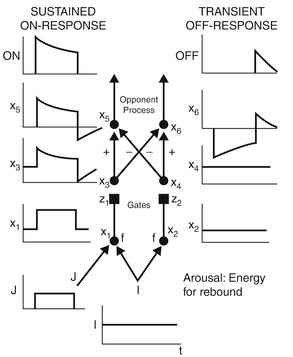# Musing on Intelligence – Part 9: Emulating Dynamic Neural Networks

Standard

In previous blog post I gave examples of how neural systems are capable of digital transmission and symbolic processing through mechanisms such as thresholding and lateral excitation and inhibition.  In this post, I will discuss the flip side: how computers can emulate dynamic behaviors of neural networks.

## Numerical integration

Computer emulation of dynamic systems is usually done through numerical integration; and Runge-Kutta-Fehlberg (RKF45) is one of the most popular methods.  It is based on the standard Runge-Kutta method but added adaptive step-size to ensure stability.  Both of these methods are for solving ordinary differential equations (ODE).

To cast neural networks as dynamic systems and solve them with RKF45 means the neural networks must be expressed as a set of ODEs.  In neural science, observed behaviors of neural networks are often described by differential equations, such as equations governing ion exchange across membranes, body potentials, synaptic conductivity, and axonal signal propagation.

## Gated dipole example

Gated dipole is a neural network model that models opponent processing, and can be used to emulate motivation and reinforcement learning.  It has an ON channel and an OFF channel, both have input,$I$; and the ON channel also has input,$J$.  The ON channel output,$x_5$ is energized at the onset of$J$; and the OFF channel output,$x_6$ is suppressed.  At the offset of$J$, however,$x_5$ is suppressed while$x_6$ is energized.Figure-1: Gated dipole.

In reinforcement learning the learning must be able to associate the salient action with the consequences.  For example, picking up a boiling pot with bare hands can lead to pain.  Putting it down will relief the pain.  In this example, the action of “picking up a boiling pot” is modeled by the onset of$J$; releasing the boiling pot is modeled by the termination of$J$.  That means the ON channel of the gated dipole can be used to activate the learning of negative reinforcement (what not to do); while the OFF channel can be used to activate the learning of positive reinforcement (what to do when it is “too hot”), where the act of relieving pain is learned.

Gated dipole is modeled by the below set of differential equations:$\dot{x}_1 = -\alpha x_1 + S_1$$\dot{x}_2 = -\alpha x_2 + S_2$$\dot{z}_1 = A (B - z_1) - S_1 z_1$$\dot{z}_2 = A (B - z_2) - S_2 z_2$$\dot{x}_3 = -\varepsilon x_3 + \zeta (x_1 - \Gamma)^+ z_1$$\dot{x}_4 = -\varepsilon x_4 + \zeta (x_2 - \Gamma)^+ z_2$$\dot{x}_5 = -\eta x_5 + \kappa (x_3 - x_4)^+$$\dot{x}_6 = -\eta x_6 + \kappa (x_4 - x_3)^+$

Note that at steady-state, i.e.,$\dot{x}_1 = 0$ and$\dot{x}_2 = 0$, and with$\alpha = 1$, the first two equations reduced to$x_1 = S_1$ and$x_2 = S_2$.  Similarly, at steady-state,$z_1 = \frac{A B}{A + S_1}$, and$z_2 = \frac{A B}{A + S_2}$.  If$\varepsilon = 1$,$\zeta = 1$, and$\Gamma = 0$, then we have$x_3 = S_1 z_1$$x_4 = S_2 z_2$.  Apply the same to the ODE for$\dot{x}_5$ and$\dot{x}_6$, we have, at steady-state,$x_5 = S_1 z_1 - S_2 z_2$, and$x_6 = -x_5$.

While the gated dipole has a steady-state solution if its inputs persists for a long period of time, the transient component of the solution is perhaps more important.  For instance, the OFF channel output, shown at the top of this article, is entirely transient.  Note that if transient behavior of gated dipole is not modeled, one would not see the OFF channel signal.

## Benefits of numerical integration

Use of numerical integrator to model neural network has some practical benefits.  First, it is universal–that means it is applied over all neural networks in the system.  The only thing users must provide is the differential equations.  Second, numerical integration can be real-time or accelerated.  Since the calculation is repeated with time stepping, the step size can be made to match the actual wall time, or it can be accelerated.  Third, large-scale neural networks can be easily partitioned among many processors.  Each tightly-coupled neural network can run on separate computing platforms, and broadcast their outputs to other neural networks.  Finally, transient behaviors, which is important in biological systems, can be modeled in artificial intelligence systems.  It is entirely conceivable that intelligent systems can be constructed by functionally partitioned interconnecting neural networks, much like the human brains.  The challenge now is determining salient behaviors for intelligence and model them with differential equations.

Starting the next blog I will begin to tie all the pieces discuss so far to form a system.